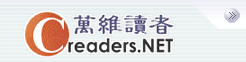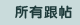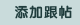设万维读者为首页广告服务技术服务联系我们关于万维简体繁体手机版
 首页 新闻 论坛 博客 视频 分类广告 购物版主：
 五 味 斋 茗香茶语 天下论坛 竞技沙龙 彩虹之约 摄友部落 诗词歌赋 七荤八素 高山流水 海 二 代 教育学术 笑林之声 健康生活 史地人物 军事天地 跨国婚姻 恋恋风尘 灵机一动 股市财经 加国移民 流行前线 新 大 陆 影视娱乐 焦点房谈 我爱我车 美国移民
 万维读者网 > 灵机一动 > 帖子
 车五进二:证明连续N个正整数的乘积能被N!整除 送交者: 侠行天涯 2012年04月17日18:20:07 于 [灵机一动] 发送悄悄话 证明连续N个正整数的乘积能被N!整除车五进二Let p be any prime number between 1 and n.In n!, there are [n/p] consecutive multiples p, where [x] is the greatest integer less than or equal to x.Therefore the number of factors of p in n! is [n/p] + [n/(p^2)] + [n/(p^3)]...For N consecutive numbers, by the pigeon hole theorem, there are at least [n/p] consecutive multiples of p, therefore the number of factors of p in the product is at least [n/p] + [n/(p^2)] + [n/(p^3)]..., hence divisible by the factors of p in n!.Since this applies to all the prime factors of n!, therefore K(K+1)...(K+N-1) is divisible by n!.
 0%(0)
 0%(0).ccc{width:675px;display:block;white-space:nowrap;overflow:hidden;float:left;-o-text-overflow: ellipsis;text-overflow:ellipsis;}实用资讯
 一周点击热帖 更多>>
 一周回复热帖
 历史上的今天：回复热帖
 2011: 给《常青人生 给》的品河蟹 焗枸杞主人 2011: 刚看到一副派，桥牌运气论的绝佳证明。 2010: 有理柯西序列定义无理数问题 2009: 简单系统可靠性问题